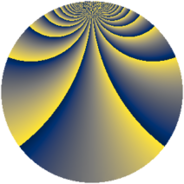# Properties

 Label 1045.2.bsLevel $1045$ Weight $2$ Character orbit 1045.bs Rep. character $\chi_{1045}(172,\cdot)$ Character field $\Q(\zeta_{20})$ Dimension $864$ Sturm bound $240$

# Related objects

## Defining parameters

 Level: $$N$$ $$=$$ $$1045 = 5 \cdot 11 \cdot 19$$ Weight: $$k$$ $$=$$ $$2$$ Character orbit: $$[\chi]$$ $$=$$ 1045.bs (of order $$20$$ and degree $$8$$) Character conductor: $$\operatorname{cond}(\chi)$$ $$=$$ $$55$$ Character field: $$\Q(\zeta_{20})$$ Sturm bound: $$240$$

## Dimensions

The following table gives the dimensions of various subspaces of $$M_{2}(1045, [\chi])$$.

Total New Old
Modular forms 992 864 128
Cusp forms 928 864 64
Eisenstein series 64 0 64

## Trace form

 $$864 q - 4 q^{3} - 4 q^{5} - 10 q^{7} + O(q^{10})$$ $$864 q - 4 q^{3} - 4 q^{5} - 10 q^{7} + 8 q^{11} - 96 q^{12} - 40 q^{15} + 200 q^{16} - 10 q^{17} - 16 q^{20} - 8 q^{22} + 8 q^{25} - 48 q^{26} - 4 q^{27} - 120 q^{28} - 80 q^{30} + 32 q^{31} - 104 q^{33} + 104 q^{36} + 36 q^{37} + 24 q^{42} + 48 q^{45} - 160 q^{46} + 16 q^{47} + 136 q^{48} - 80 q^{51} - 80 q^{52} + 20 q^{55} + 80 q^{56} + 96 q^{58} - 136 q^{60} + 80 q^{61} - 300 q^{62} + 50 q^{63} + 104 q^{66} - 80 q^{67} + 40 q^{68} - 80 q^{70} - 72 q^{71} - 300 q^{72} - 120 q^{73} - 16 q^{75} + 14 q^{77} + 80 q^{78} - 180 q^{80} + 104 q^{81} - 80 q^{82} - 80 q^{86} - 308 q^{88} - 8 q^{91} + 96 q^{92} + 36 q^{93} - 10 q^{95} + 120 q^{96} - 28 q^{97} + O(q^{100})$$

## Decomposition of $$S_{2}^{\mathrm{new}}(1045, [\chi])$$ into newform subspaces

The newforms in this space have not yet been added to the LMFDB.

## Decomposition of $$S_{2}^{\mathrm{old}}(1045, [\chi])$$ into lower level spaces

$$S_{2}^{\mathrm{old}}(1045, [\chi]) \cong$$ $$S_{2}^{\mathrm{new}}(55, [\chi])$$$$^{\oplus 2}$$